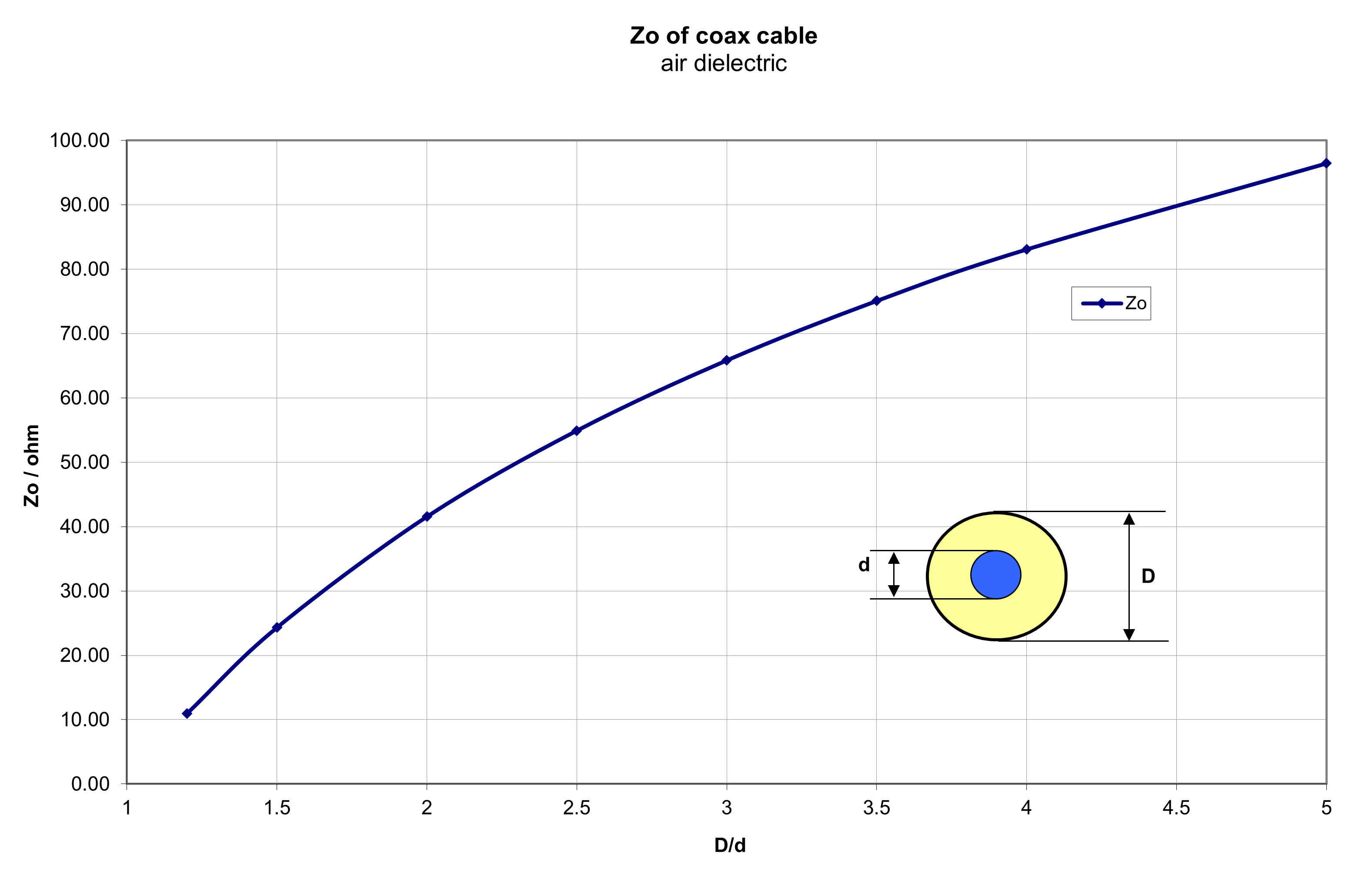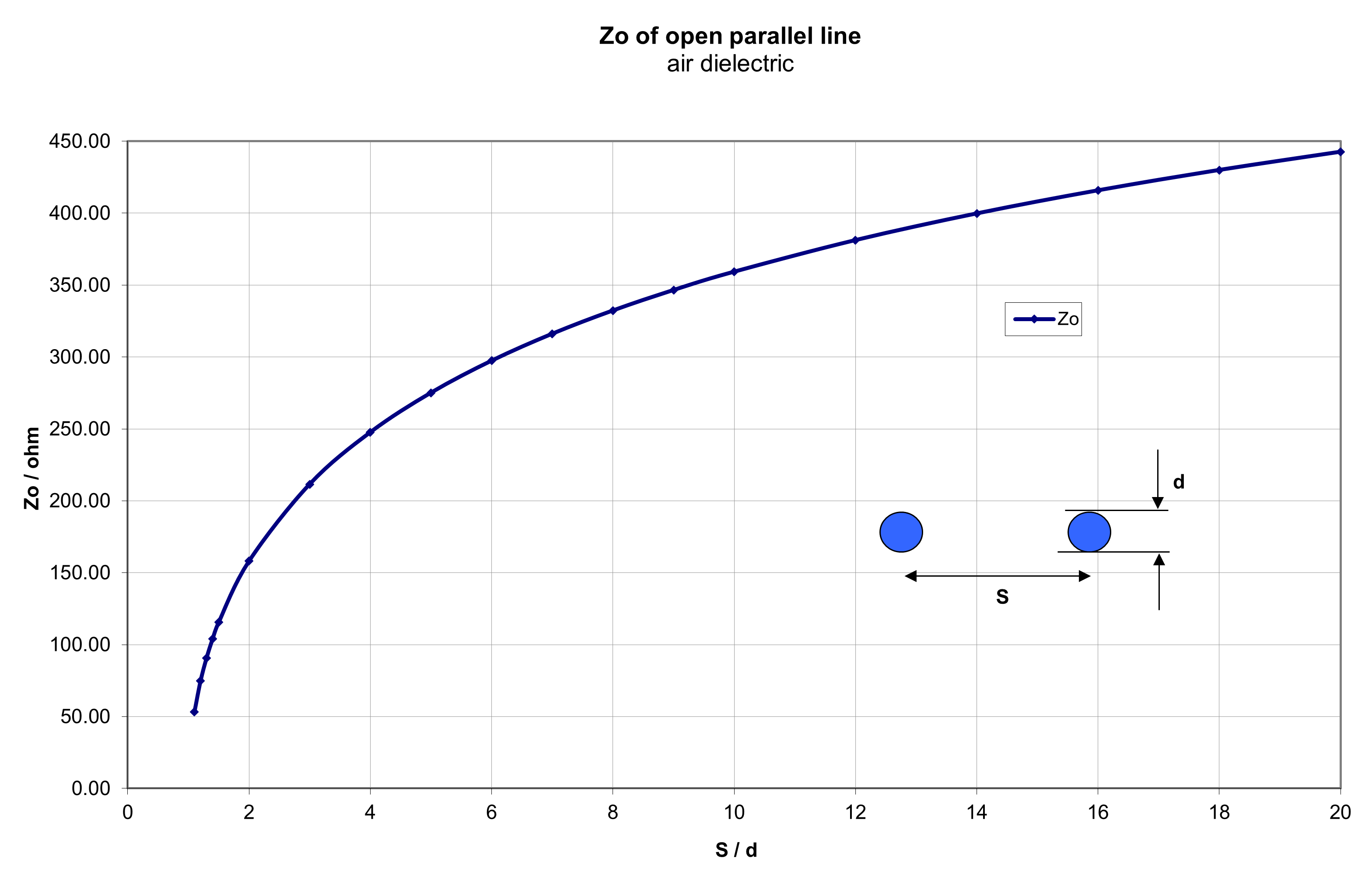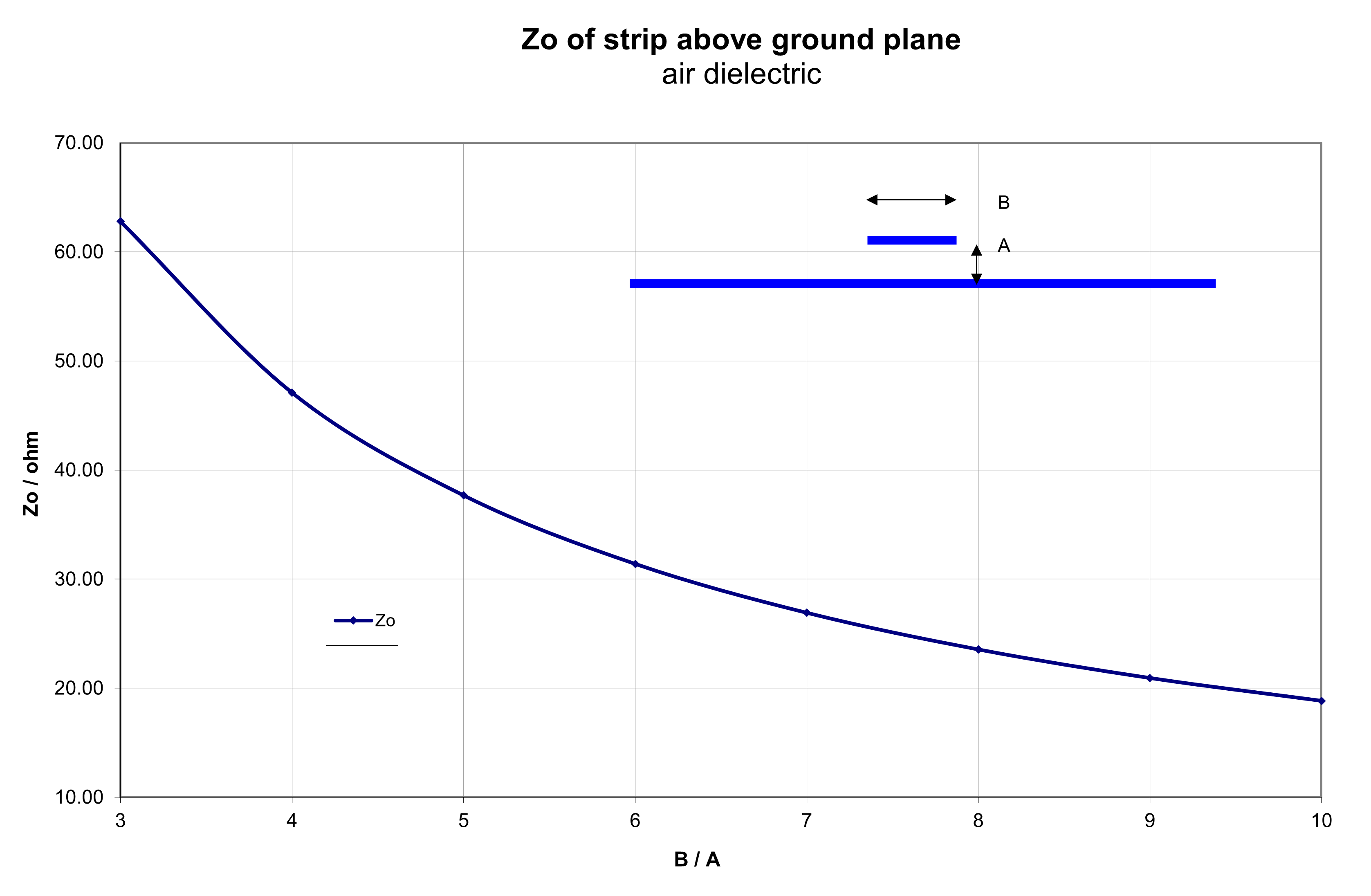### Transmission line calculators 2

My project number 25 from 2010 about transmission lines was originally in Excel format. Now in 2021 I have done here the same with html and Java scripts, which i hope is easier to use.

#### Coaxial cableZo / Ω = 138 * log(D/d) / SQRT(ε)

Give inner diameter D of the outer conductor. D and d in same units.

❶.

Give outer diameter d of the inner conductor

❷.

Give dielectric constant ε of the media between conductors, for air it is 1.

❸.

Cable impedance is:

Cable capacitance [far end open] is:   ;     C / pF/m = 24.1 * ε / log(D/d)

Cable inductance [far end shorted] is:   ;     L / μH/m = 0.459 * log(D/d)

Capacitance and inductance valid only, when cable length is much less than a wavelength.

Velicity factor v is:   ;       v = 1 / SQRT(ε)#### Parallel line cableZo / Ω = 120 * ACOSH(S/d)

Give wire distance S. S and d in same units.

❶.

Give wire diameter d

❷.

Cable impedance is:#### Parallel strip-lineZo / Ω = 377 * a / b ,   where a much smaller than b.

Give strip distance a.   a and b in same units.

❶.

Give strip width b

❷.

Cable impedance is:

#### Strip above ground planeZo / Ω = 188.4 * a / b ,   where A much smaller than B.

Give strip distance A.   A and B in same units.

❶.

Give strip width B, shall be much bigger than A

❷.

Cable impedance is:Pekka Ketonen 17.5.2021